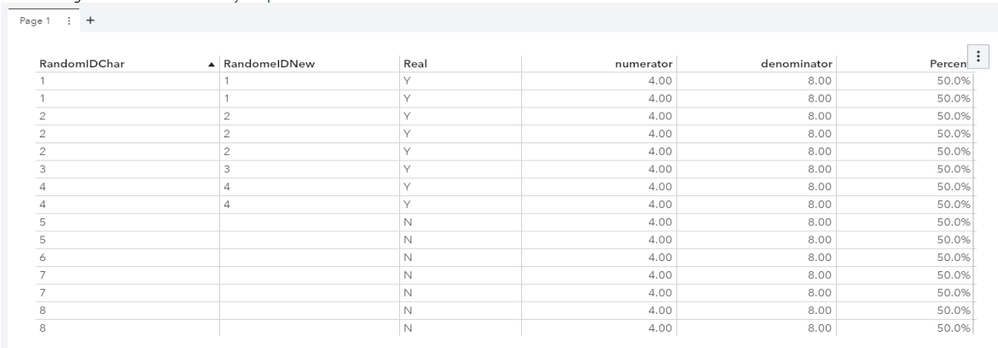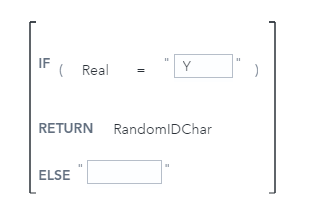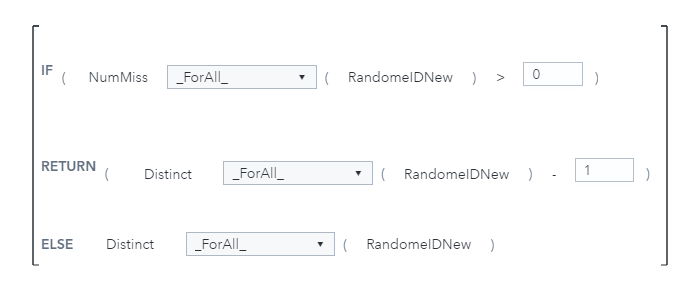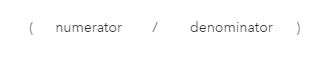DavidPhillips2
Rhodochrosite | Level 12

## Distinct Subpopulation Divided by Distinct Population

I’m trying to display the distinct count of a population where a particular condition in the record is true.  My text in the edit calculated item of VA Report Editor is:

IF ( 'REAL Numeric'n = 1 )

RETURN Distinct [_ByGroup_] ('Random ID'n[Formatted])

ELSE 0

The goal is to produce the distinct count of the student population where a condition is true divided by the distinct count of the student population and show it as a static or sometimes referred to as a key value.

For some reason, the result of this is 0.

Since this is for a dashboard I cannot just display both numbers in tabular format.

1 ACCEPTED SOLUTION

Accepted Solutions

## Re: Distinct Subpopulation Divided by Distinct Population

David,

I was able to get it to work. This is what my sample data looked like.  It started off with just RandomIDChar and Real.   I assume that your goal is to get one value and add it to a Key Value object.Calculations:

Step #1: Create your new character data item based on your filter criteria:

Name: RandomIDNew
Calculation:Step #2: Numerator: (Note: NumMiss will calculate the number of items that are missing. If there are any missing, then we need to subtract that one distinct count from the resulting distinct count calculation)Step #3:  Denominator:Step #4:  Create Percent  (Note:  step 2,3 & 4 could all be just one calculation)3 REPLIES 3DavidPhillips2
Rhodochrosite | Level 12

## Re: Distinct Subpopulation Divided by Distinct Population

With this syntax the is = 1 is being compared to the aggregated set, so that logic doesn't work.

I've been playing with the notmissing function to see if there is a way to remove the missing values.

The if only has a if else function, if I could drop the else it would work.DavidPhillips2
Rhodochrosite | Level 12

## Re: Distinct Subpopulation Divided by Distinct Population

I'm investigating options involving subtracting out the values that would be missing.  Investigating functions like nummiss.

## Re: Distinct Subpopulation Divided by Distinct Population

David,

I was able to get it to work. This is what my sample data looked like.  It started off with just RandomIDChar and Real.   I assume that your goal is to get one value and add it to a Key Value object.Calculations:

Step #1: Create your new character data item based on your filter criteria:

Name: RandomIDNew
Calculation:Step #2: Numerator: (Note: NumMiss will calculate the number of items that are missing. If there are any missing, then we need to subtract that one distinct count from the resulting distinct count calculation)Step #3:  Denominator:Step #4:  Create Percent  (Note:  step 2,3 & 4 could all be just one calculation)Discussion stats
• 3 replies
• 589 views
• 0 likes
• 2 in conversation